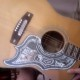### a couple of good exercises at random before breakfast

in one sense, “textbook” exercises.
but in the usual sense, not.
i made up these “exercises” for myself,
but they sure as heck involve a *textbook*.
just now… very much in my usual way…
i made some pencil notes on a textbook page.

there was even a “warm-up”.
my *first* marks on that page…
“page 43”, let’s say… were to
scratch over the first “of” of

In number theory, the function of given in Theorem 2.2 would be called an ordered composition of k with n parts, because it gives a list of n numbers that add up to k.
and replace it with “f“.

because theorem 2.2, as it turns out,
tells us that

Theorem 2.2. The number of functions f from a set${x_1, x_2, \ldots x_n}$ to the nonnegative integers such that$\sum_{i=1}^n f(x_i) = k$ is C(n + k – 1; k).

or, in other words, that$\sharp \{ f \mid f: \{x_i\}_{i=1}^{n} \rightarrow {\Bbb N}, \sum_{i=1}^n f(x_i) = k\} ={{n+k-1}\choose k}$.

and that was, if you will, “exercise 1”:
“restate theorem 2.2 wordlessly”.
this code now appears in the (generous)
margin of page 43 (with the unimaginative
label “THEOREM 2.2”).

exercise 2?
well, the$\sum_{i=1}^n f(x_i) = k$
bit appears *twice* on page 43
centered on a line of its own
(in a “display”, in other words).
so next to the first such display
i wrote out
(
f TAKES
n VALUES
TO k
)
… where the parens “(“,”)” indicate
big ol’ *handwritten* parens
bracketing the whole “block”
of handwritten “f takes n
values adding up to k” thing;
it fills the “display” space
(to the right of the displayed code)
rather nicely from top to bottom.

so the first “exercise” was of the form:
“translate from english into code”
and the second was of the form
“translate from code into english”.

“write it up in$\TeX$” will here
be considered beyond the scope of our
post
… it *is* time for breakfast…

i just couldn’t wait to observe here that such
“translating”, back-and-forth, code-to-language,
language-to-code is a *large part* of “doing math”.
throw in code-to-graph (and back) and get even more.
venn-diagrams back-and-forth to truth tables.
(hey, look. “isomorphisms”!)

anyhow. we’re *always* doing suchlike exercises.
trouble is. what-you-mean-“we”?

*feed* me!

1.vlorbik